## 哲学基础：因果推断何以成为可能？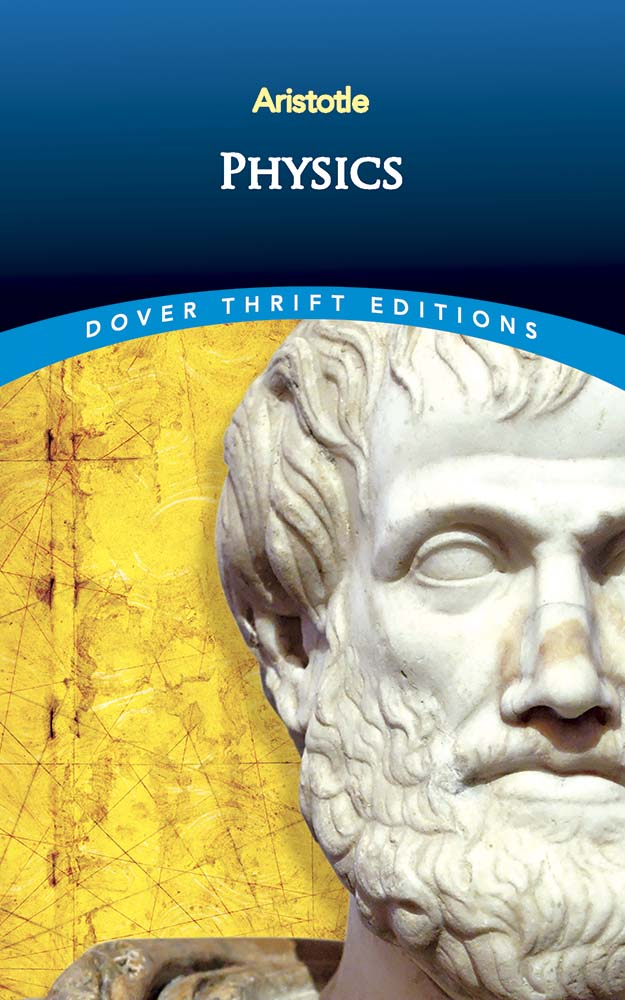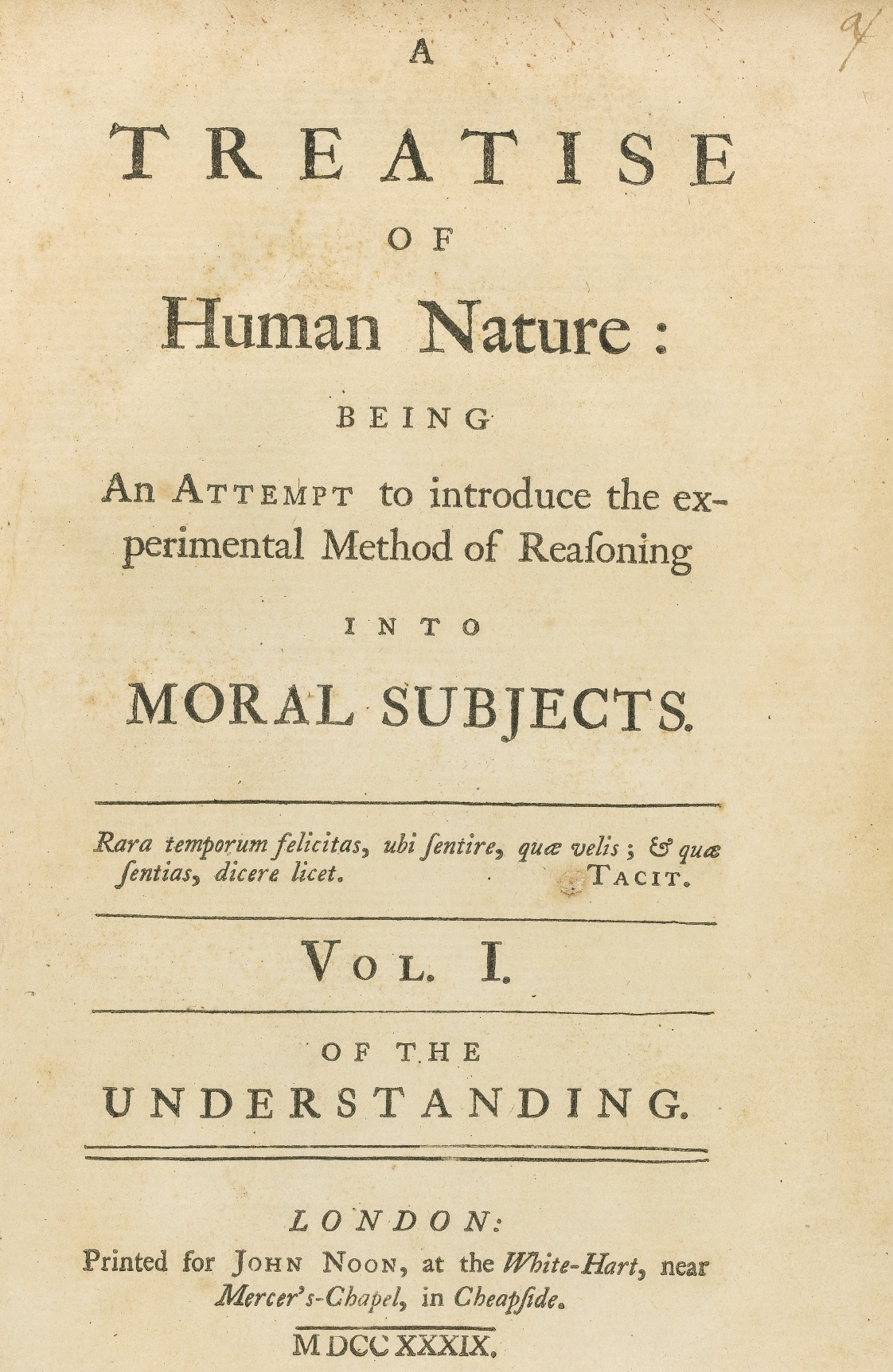## 统计学中“哥白尼式的革命”：内曼的“潜在结果”模型

1923 年，耶日• 内曼（Jerzy Neyman, 1894-1981） 还是波兰华沙大学的博士生，他的毕业论文是“概率论在农业实验中的应用”3。在这篇论文中，他提出了用于因果推断的“潜在结果”（potential outcomes）的数学模型，并将它和统计推断结合起来。他的想法非常自然，数学结构也很简单。下面简单地回顾一下。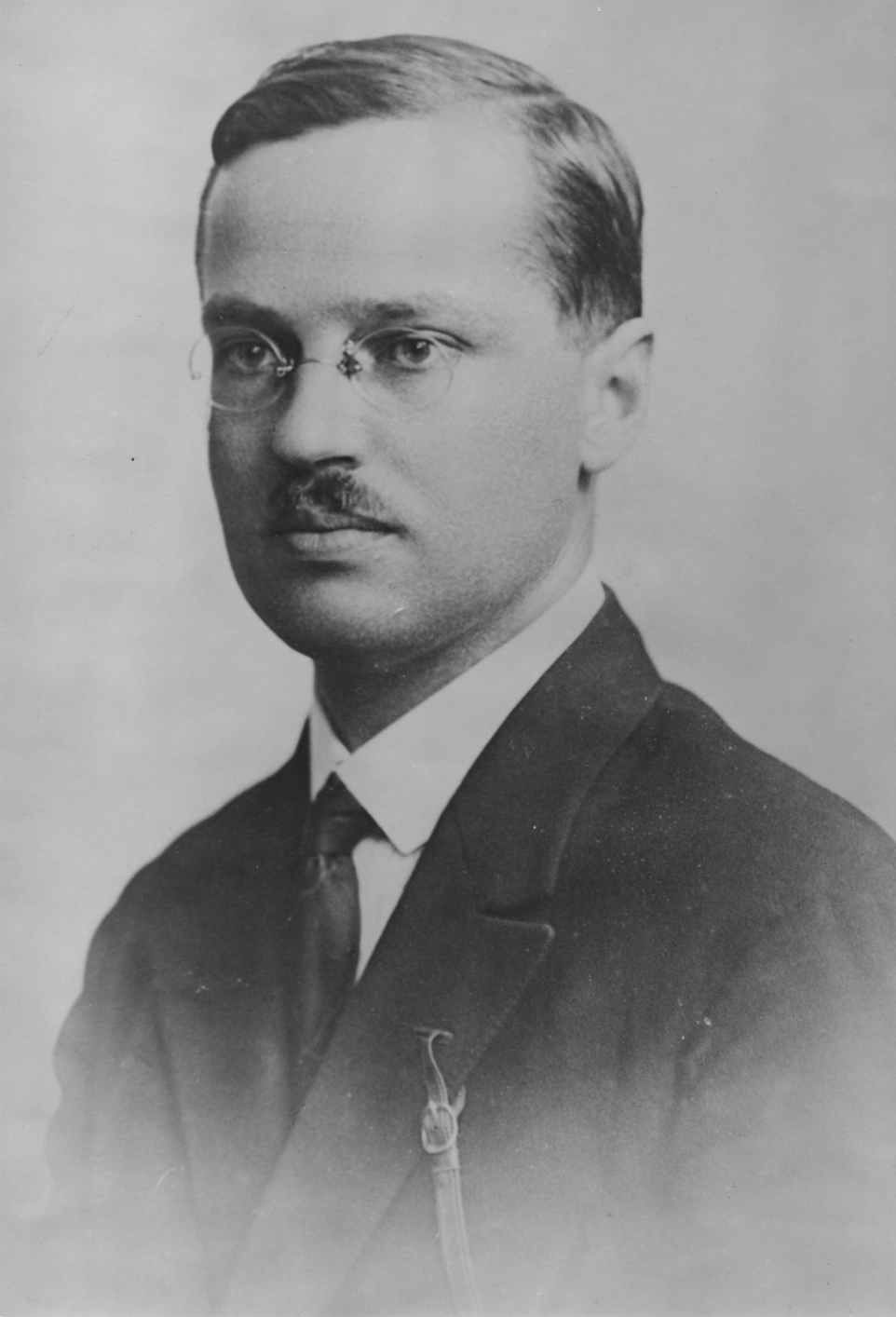$$\tau = \frac{1}{n} \sum_{i=1}^n \tau_i$$

$$Y_i = Y_i (Z_i) = Z_i Y_i (1) + (1 - Z_i)Y_i(0).$$

$$\hat{\tau} = \frac{1}{n_1} \sum_{i:Z_i=1} Y_i - \frac{1}{n_0} \sum_{i:Z_i=0} Y_i .$$

## 统计学的拓荒者：鲁宾关于观察性研究中的因果推断的研究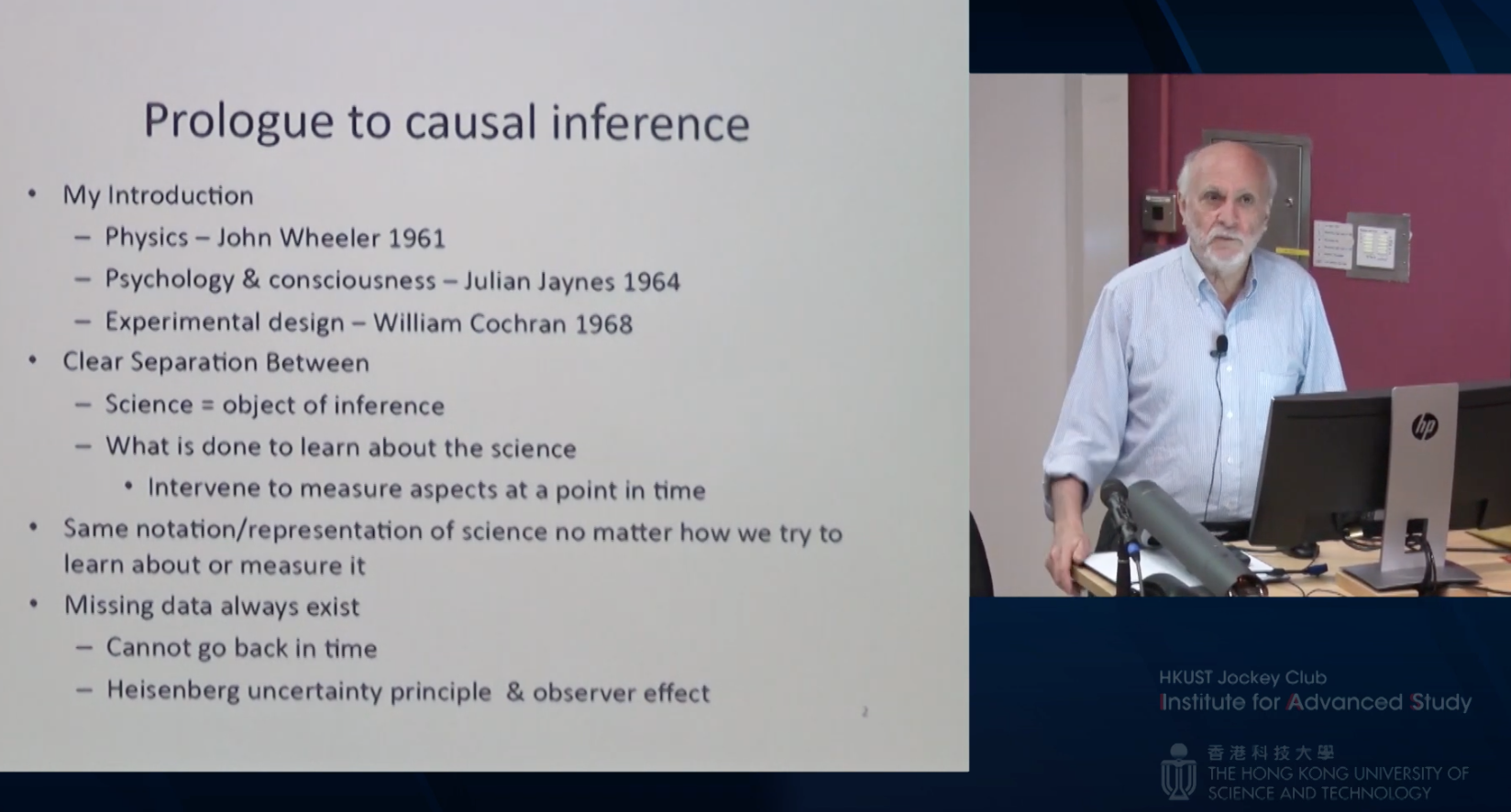• $Z_i$: 个体 $i$ 吸烟与否的指示变量；
• $Y_i$: 个体 $i$ 是否得肺癌的指示变量；
• $X_i$: 个体 $i$ 的年龄、性别、教育、收入、家庭病史等等，统计学中称它们为协变量（covariates）。

$$\tau = E\{ Y_i (1) - Y_i (0) \}.$$

\begin{aligned} \tau &= \sum_x \{E(Y_i(1) \mid X_i=x) − E(Y_i (0) \mid X_i = x)\}P(X_i = x)\\ &= \sum_x \{E(Y_i(1) \mid Z_i = 1, X_i = x) − E(Y_i (0) \mid Z_i = 0, X_i = x)\}P(X_i = x)\\ &= \sum_x \{E(Y_i \mid Z_i = 1, X_i = x) − E(Y_i \mid Z_i = 0, X_i = x)\}P(X_i = x). \end{aligned}

$$\tau = E\left\{ \frac{Z_i Y_i}{e(X_i)} \right\} - E\left\{ \frac{(1-Z_i)Y_i}{1 - e(X_i)} \right\},$$

Rosenbaum 和鲁宾的这篇文章是 Biometrika 这个杂志创刊以来引用率最高的两篇文章之一7。在它发表后的三十多年里，引起了很多理论统计学家和应用统计学家的兴趣，他们提出了很多推广的、更加精致的理论和方法，这些理论和方法被用在流行病学、经济学、政治科学等诸多学科的研究中。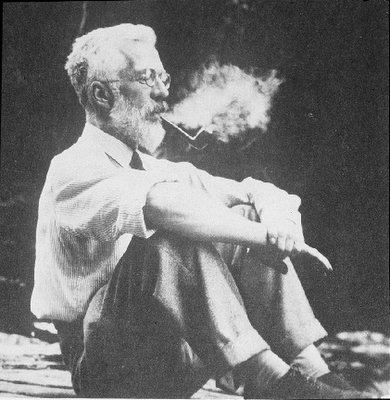## 人工智能的“因果革命”：珀尔对图模型的因果解释

$$P(X) = \prod_{j=1}^p P(X_j\mid\text{pa}_j).$$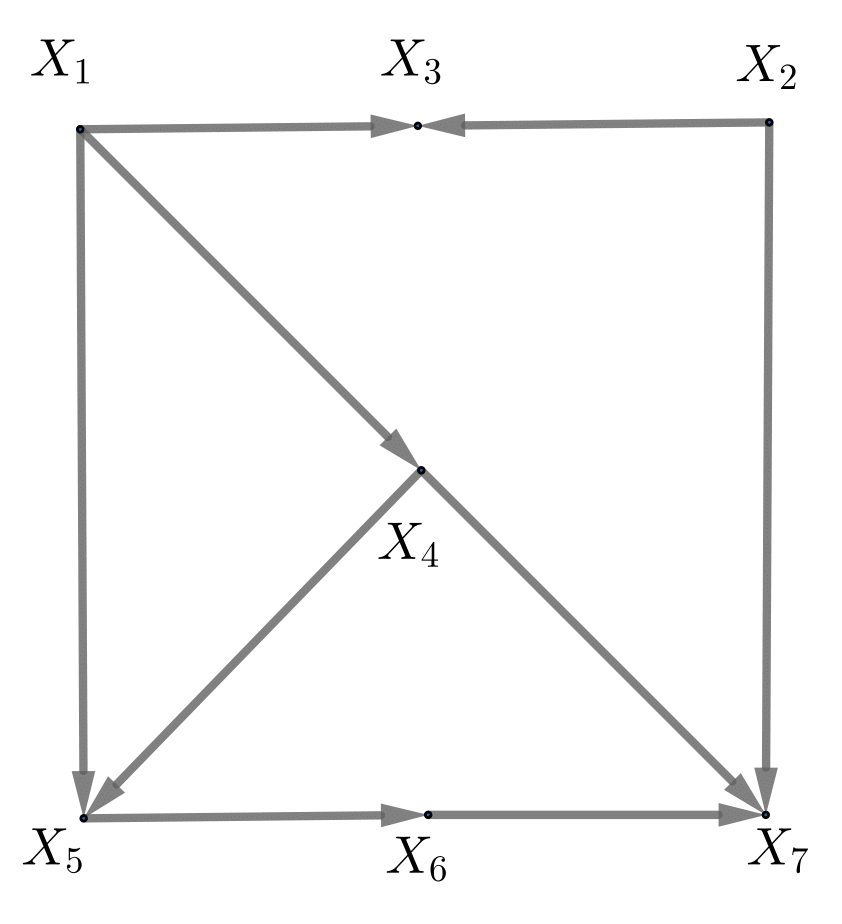\begin{aligned} P(X) &= P(X_1) \cdot P(X_2) \cdot P(X_3\mid X_1,X_2) \cdot P(X_4\mid X_1) \\ & \cdot P(X_5\mid X_1,X_4) \cdot P(X_6\mid X_5) \cdot P(X_7\mid X_2, X_4, X_6). \end{aligned}

$$P(X_1=x_1, \cdots, X_p=x_p\mid \text{do}(X_j=x_j^{'})) = \frac{P(X_1=x_1, \cdots, X_p=x_p)}{P(X_j=x_j\mid \text{pa}_j)} I(x_j=x_j^{'})$$

$$P(X_7 = x_7 \mid \text{do}(X_5 = 1)), \quad P(X_7 = x_7 \mid \text{do}(X_5 = 0)),$$

$$E(X_7\mid \text{do}(X_5=1)), \quad E(X_7\mid \text{do}(X_5=0)),$$

$$P(X_7=x_7\mid \text{do}(X_5=1)) \neq P(X_7=x_7\mid X_5=1)$$

\begin{aligned} P(X\mid \text{do}(X_5=1)) &= P(X_1) \cdot P(X_2) \cdot P(X_3\mid X_1,X_2) \cdot P(X_4\mid X_1,X_2,X_3) \\ & \cdot P(X_6\mid X_5) \cdot P(X_7\mid X_2, X_4, X_6) \cdot I(X_5=1). \end{aligned}

### 后门准则

$$X_5 \leftarrow X_4 \rightarrow X_7,\\ X_5 \leftarrow X_1 \leftarrow X_4 \rightarrow X_7,$$

$$P(X_7=x_7\mid \text{do}(X_5=1)) = \sum_{x_4} P(X_7=x_7\mid X_4=x_4, X_5=1)P(X_4=x_4)$$

\begin{aligned} \tau &= E(X_7 \mid \text{do}(X_5 = 1)) − E(X_7 \mid \text{do}(X_5 = 0)) \\ &= \sum_{x_4} \{E(X_7 \mid X_4 = x_4, X_5 = 1) − E(X_7 \mid X_4 = x_4, X_5 = 0)\} P (X_4 = x_4). \end{aligned}

### 前门准则

\begin{aligned} &P(X_7=x_7\mid \text{do}(X_5=1)) \\ = & \sum_{x_6} P (X_6 = x_6 \mid X_5 = 1) \sum_{x_5} P (X_7 = x_7 \mid X_5 = x_5, X_6 = x_6)P (X_5= x_5). \end{aligned}

(a) $X_5$$X_6$ 的因果作用是可以识别的，因为他们之间没有后门路径；

(b) $X_6$$X_7$ 的因果作用是可以识别的，因为他们的后门路径被 $X_5$ 阻断了；

(c) $X_5$$X_7$ 的因果作用仅仅通过 $X_6$ 产生，因此，$X_5$$X_7$ 的因果作用可以理解成 $X_5$$X_6$ 的因果作用和 $X_6$$X_7$ 的因果作用的“乘积” 。

## 中国因果推断的研究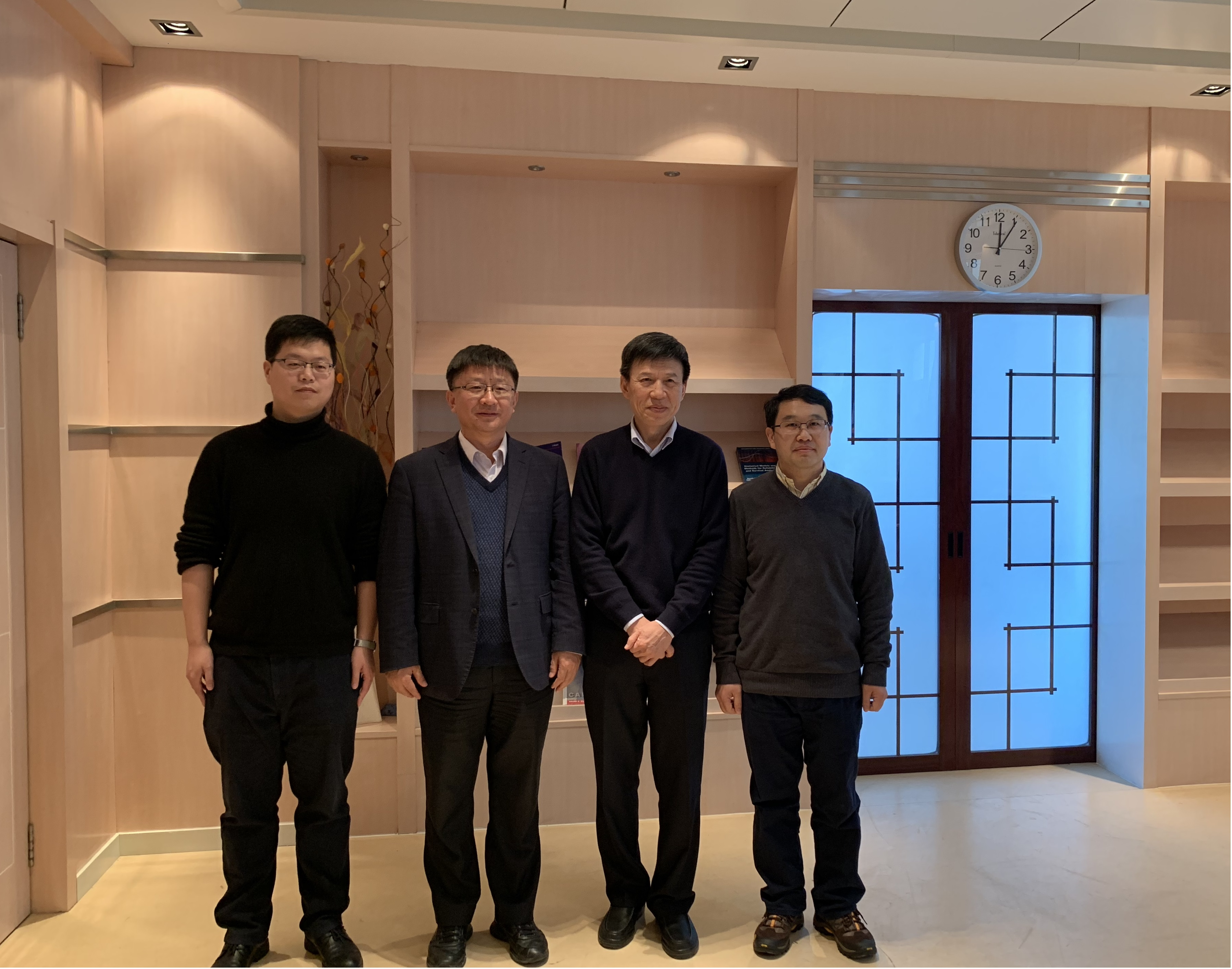### 替代指标悖论和准则、统计和因果关系的传递性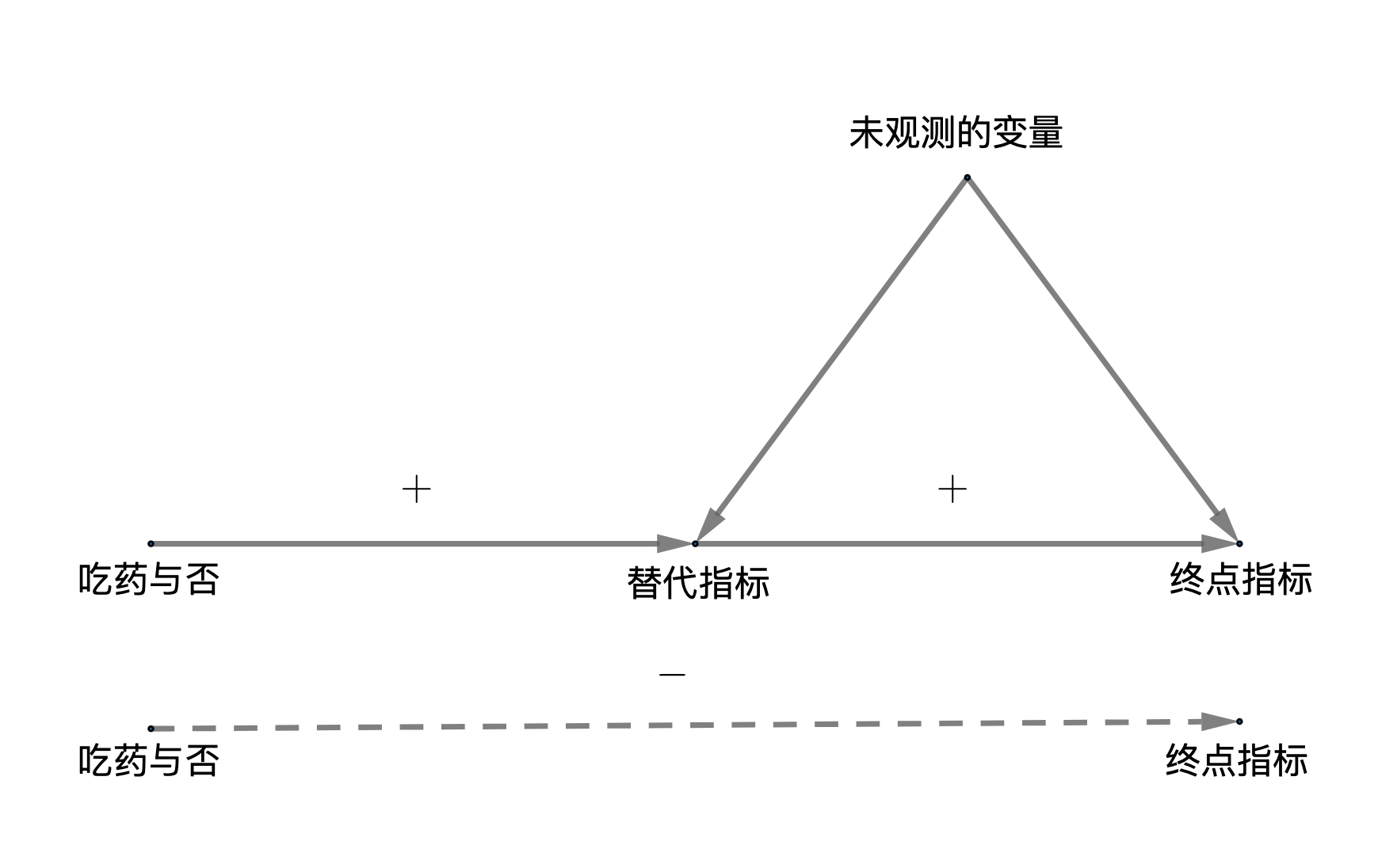## 注释

1. A. Gelman and A. Vehtari, What are the most important statistical ideas of the past 50 years? 见https://arxiv.org/abs/2012.00174。 第一作者曾获得年轻统计学家的最高奖 COPSS 奖章。

2. “[T]he sole end of science is the honor of the human mind.” —— Carl Jacobi（卡尔• 雅可比）

3. 内曼的论文是用波兰语写成的。1990 年，D. M. Dabrowska 和 T. P. Speed 将论文翻译成英文，题目是 On the Applications of the Theory of Probability to Agricultural Experiments，发表于 Statistical Science。潜在结果的基本想法也许在历史中早就产生了，但是将它数学化、且正式地用于统计学，内曼的文章是首次。内曼是现代统计学的奠基人之一，他对假设检验、置信区间、抽样调查和实验设计等领域的研究，成为现代统计学的标准范式。我国概率论和数理统计学的先驱许宝騄教授是内曼在英国指导的学生之一。

4. 这方面的文献综述是：Li, X. and Ding, P. (2017). General forms of finite population central limit theorems with applications to causal inference. Journal of the American Statistical Association, 112, 1759-1769。

5. 见内曼的传记：C. Reid (1982), Neyman - From Life。注意，哥白尼和内曼都是波兰人。

6. 另外一位受内曼影响的是计量经济学家 Trygve Haavelmo。他是在计量经济学中讨论因果推断的先驱。他曾在1989 年诺贝尔经济学奖的获奖感言中谈及内曼对他的影响: https://www.nobelprize.org/prizes/economic-sciences/1989/haavelmo/facts/

7. 文章是 Rosenbaum and Rubin (1983) The central role of the propensity score in observational studies for causal effects, Biometrika, 70, 41-55。在纪念 Biometrika 第一百期的时候，这篇文章的引用数在该杂志排名第二；参看 Titterington (2013) Biometrika highlights from volume 28 onwards, Biometrika, 100, 17-73。截至写作本文的时候，Google Scholar 显示这篇文章已经被引用了28392 次，已经超越了之前引用最高的文章 Liang and Zeger (1986) Longitudinal data analysis using generalized linear models, Biometrika, 73, 13–22（Google Scholar 显示引用了18345 次）。这种改变，反映了近十年来，因果推断的研究在学术界的极端活跃性。另外，Biometrika 创刊于 1901 年，是最早的理论统计杂志之一。

8. 第一本是 Rubin (2006) Matched Sampling for Causal Effects。第二本是 Imbens and Rubin (2016) Causal Inference for Statistics, Social, and Biomedical Sciences: An Introduction。两书均由剑桥大学出版社出版。

9. 比如 A. P. Dempster 就用一个无向图来表示联合正态分布中的条件独立性：给定其他变量，如果两个变量条件独立， 那么他们之间的边不存在。他的文章是: Dempster, A.P. (1972) Covariance selection. Biometrics, 157-175。

10. 珀尔的开创性文章是：Pearl (1995) Causal diagrams for empirical research. Biometrika, 82, 669-688.他的专著是： Pearl (2009) Causality: Models, Reasoning and Inference, 剑桥大学出版社。

11. 此书英文原名是 A System of Logic，直接翻译过来是《一个逻辑体系》，严复先生认为“逻辑学”就是中国的“名学”， 这一学派兴起于先秦，代表人物有公孙龙等。这本书在英语世界产生过很深远的影响，其中五条“穆勒方法”总结了归纳推理中，获得因果知识的一些准则。严复先生是北京大学从“京师大学堂”更名后的第一任校长，也曾任复旦大学校长。

12. 原书这章的题目是“On observation and experiment”。按照现在的习惯，“experiment”统一翻译成“实验”。前面用到的“临床试验”对应着“clinical trial”。“实验”和“试验”的意思似乎差别不大；中文英文皆如此。

13. Geng (1992) pp. 585-593; Geng and Asano (1993), pp. 741–747; Guo and Geng (1995), pp. 263-267; Geng, Guo and Fung (2002), pp. 3-15; Ma, Xie and Geng (2006), pp. 127-133。

14. Prentice 曾获得年轻统计学家的最高奖 COPSS 奖章，终身成就奖“费希尔讲座”，他是美国医学院院士。鲁宾是因果推断的奠基人之一，曾获得终身成就奖“费希尔讲座”，美国科学院院士。Lauritzen 是英国皇家学会院士。

15. Chen, Geng and Jia (2007), pp. 911-932; Ju and Geng (2010), pp. 129-142; Jiang, Ding and Geng (2016) pp. 829-848。

16. 文章是 VanderWeele (2013) Surrogate measures and consistent surrogates. Biometrics, 69, 561-565。 VanderWeele 曾获COPSS 奖章。

17. https://terrytao.wordpress.com/2014/06/05/when-is-correlation-transitive/

18. Xie and Geng (2008), pp. 459-483; Ma, Xie and Geng (2008), pp. 2847-2880; He and Geng (2008), pp. 2523-2547; Liu et al. (2020)。

19. 本希奥的文章 Towards Causal Representation Learning 出现在 https://arxiv.org/abs/2102.11107